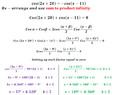# Solve trig equation cos(2x+20)=-cos(x-11)

• MHB
laprec
Kindly assist with this question:
Determine the general solutions cos(2x+20)=-cos(x-11)

Last edited:

skeeter
$\cos(2x+20)+\cos(x-11)=0$

using sum to product identity

$2\cos\left(\dfrac{3x+9}{2}\right)\cos\left(\dfrac{x+31}{2}\right) =0$

setting each cosine factor equal to zero yields

$\dfrac{3x+9}{2} = \dfrac{\pi}{2}(2k+1)$ where $k \in \mathbb{Z}$

$\dfrac{x+31}{2} = \dfrac{\pi}{2}(2k+1)$ where $k \in \mathbb{Z}$

if the arguments of the cosine functions are in degrees, then

$\dfrac{3x+9}{2} = 90(2k+1)$ where $k \in \mathbb{Z}$

$\dfrac{x+31}{2} = 90(2k+1)$ where $k \in \mathbb{Z}$

finish it

Last edited by a moderator:
Homework Helper
MHB
As an alternative to skeeter's method, I'd like to bring up the unit circle.
\begin{tikzpicture}[scale=3,>=stealth]
\def\angle{35}
\draw[->, help lines] (-1.2,0) -- (1.2,0);
\draw[->, help lines] (0,-1.2) -- (0,1.2);
\draw[ultra thick, blue] circle (1);
\draw[thick] (0,0) -- (\angle:1) -- ({cos(\angle)}, 0) node[below] {$\cos\theta$} -- cycle ;
\draw[thick] (0,0) -- ({180+\angle}:1) -- ({-cos(\angle)}, 0) node[below] {$-\cos\theta$} -- cycle;
\draw[thick] (0,0) -- ({180-\angle}:1) -- ({-cos(\angle)}, 0);
\draw[->] ({\angle/2}:.4) node {$\theta$} (0:.3) arc (0:\angle:.3);
\draw[->] ({(180+\angle)/2}:-.14) node {$180^\circ+\theta$} (0:.1) arc (0:{180+\angle}:.1) ;
\draw[->] ({(180-\angle)/2}:.29) node {$180^\circ-\theta$} (0:.2) arc (0:{180-\angle}:.2) ;
\end{tikzpicture}
We have an equation of the form $\cos\theta = -\cos\phi$.
Given a $\theta$, for $\cos\theta$ to be equal to the opposite of another cosine, we can see that the other angle must either be $180^\circ-\theta$ or $180^\circ+\theta$. And we may have to add a multiple of $360^\circ$, which is the period of the circle.

So:
\begin{array}{lcl}
\cos(2x+20^\circ)=-\cos(x-11^\circ) \\
2x + 20^\circ = 180^\circ - (x-11^\circ) + 360^\circ k &\lor& 2x + 20^\circ = 180^\circ + (x-11^\circ) + 360^\circ k \\
3x = 171^\circ + 360^\circ k &\lor& x = 149^\circ + 360^\circ k \\
x = \frac 13\cdot171^\circ + 120^\circ k &\lor& x = 149^\circ + 360^\circ k \\
\end{array}

laprec
$\cos(2x+20)+\cos(x-11)=0$

using sum to product identity

$2\cos\left(\dfrac{3x+9}{2}\right)\cos\left(\dfrac{x+31}{2}\right) =0$

setting each cosine factor equal to zero yields

$\dfrac{3x+9}{2} = \dfrac{\pi}{2}(2k+1)$ where $k \in \mathbb{Z}$

$\dfrac{x+31}{2} = \dfrac{\pi}{2}(2k+1)$ where $k \in \mathbb{Z}$

if the arguments of the cosine functions are in degrees, then

$\dfrac{3x+9}{2} = 90(2k+1)$ where $k \in \mathbb{Z}$

$\dfrac{x+31}{2} = 90(2k+1)$ where $k \in \mathbb{Z}$

finish it
Thanks a million skeeter. Much appreciated!
I have attached complete work out based on your guidiance.

- - - Updated - - -

As an alternative to skeeter's method, I'd like to bring up the unit circle.
\begin{tikzpicture}[scale=3,>=stealth]
\def\angle{35}
\draw[->, help lines] (-1.2,0) -- (1.2,0);
\draw[->, help lines] (0,-1.2) -- (0,1.2);
\draw[ultra thick, blue] circle (1);
\draw[thick] (0,0) -- (\angle:1) -- ({cos(\angle)}, 0) node[below] {$\cos\theta$} -- cycle ;
\draw[thick] (0,0) -- ({180+\angle}:1) -- ({-cos(\angle)}, 0) node[below] {$-\cos\theta$} -- cycle;
\draw[thick] (0,0) -- ({180-\angle}:1) -- ({-cos(\angle)}, 0);
\draw[->] ({\angle/2}:.4) node {$\theta$} (0:.3) arc (0:\angle:.3);
\draw[->] ({(180+\angle)/2}:-.14) node {$180^\circ+\theta$} (0:.1) arc (0:{180+\angle}:.1) ;
\draw[->] ({(180-\angle)/2}:.29) node {$180^\circ-\theta$} (0:.2) arc (0:{180-\angle}:.2) ;
\end{tikzpicture}
We have an equation of the form $\cos\theta = -\cos\phi$.
Given a $\theta$, for $\cos\theta$ to be equal to the opposite of another cosine, we can see that the other angle must either be $180^\circ-\theta$ or $180^\circ+\theta$. And we may have to add a multiple of $360^\circ$, which is the period of the circle.

So:
\begin{array}{lcl}
\cos(2x+20^\circ)=-\cos(x-11^\circ) \\
2x + 20^\circ = 180^\circ - (x-11^\circ) + 360^\circ k &\lor& 2x + 20^\circ = 180^\circ + (x-11^\circ) + 360^\circ k \\
3x = 171^\circ + 360^\circ k &\lor& x = 149^\circ + 360^\circ k \\
x = \frac 13\cdot171^\circ + 120^\circ k &\lor& x = 149^\circ + 360^\circ k \\
\end{array}

Thanks a lot Klaas van Aarsen, the alternative method is equally helpful and insightful. Much appreciated.

#### Attachments

•mhboardsoln.PNG
13.7 KB · Views: 40
laprec
small correction ...

Thank you very much! Much appreciated!